Impact Factor 2.073
2017 JCR, Clarivate Analytics 2018

The world's most-cited Neurosciences journals

# Frontiers in Computational Neuroscience

## Original Research ARTICLE

Front. Comput. Neurosci., 23 November 2010 | https://doi.org/10.3389/fncom.2010.00145

# Time and category information in pattern-based codes

• Centro Atómico Bariloche and Instituto Balseiro, San Carlos de Bariloche, Argentina

Sensory stimuli are usually composed of different features (the what) appearing at irregular times (the when). Neural responses often use spike patterns to represent sensory information. The what is hypothesized to be encoded in the identity of the elicited patterns (the pattern categories), and the when, in the time positions of patterns (the pattern timing). However, this standard view is oversimplified. In the real world, the what and the when might not be separable concepts, for instance, if they are correlated in the stimulus. In addition, neuronal dynamics can condition the pattern timing to be correlated with the pattern categories. Hence, timing and categories of patterns may not constitute independent channels of information. In this paper, we assess the role of spike patterns in the neural code, irrespective of the nature of the patterns. We first define information-theoretical quantities that allow us to quantify the information encoded by different aspects of the neural response. We also introduce the notion of synergy/redundancy between time positions and categories of patterns. We subsequently establish the relation between the what and the when in the stimulus with the timing and the categories of patterns. To that aim, we quantify the mutual information between different aspects of the stimulus and different aspects of the response. This formal framework allows us to determine the precise conditions under which the standard view holds, as well as the departures from this simple case. Finally, we study the capability of different response aspects to represent the what and the when in the neural response.

## 1 Introduction: Patterns in the Neural Response

Sensory neurons represent external stimuli. In realistic conditions, different stimulus features (for example, the presence of a predator or a prey) appear at irregular times. Therefore, an efficient sensory system should not only represent the identity of each perceived stimulus, but also, its timing. Colloquially, qualitative differences between stimulus features have been called the what in the stimulus, whereas the temporal locations of the features constitute the when. Spike trains can encode both the what and the when, for example, as a sequence of spike patterns. This idea constitutes a standard view (Theunissen and Miller, 1995; Borst and Theunissen, 1999; Krahe and Gabbiani, 2004), where the timing of patterns indicates when stimulus features occur, while the pattern identities tag what stimulus features happened (Martinez-Conde et al., 2002; Alitto et al., 2005; Oswald et al., 2007; Eyherabide et al., 2008). The information provided by the distinction between different spike patterns is here called category information. In the same manner, the information transmitted by the timing of spike patterns is here called time information. According to the standard view, the category and the time information represent the knowledge of the what and the when in the stimulus, respectively. In this work, we address the conditions under which these assumptions hold, as well as departures from the standard view.

Many studies have shown the ubiquitous presence of patterns in the neural response. The patterns can be, for instance, high-frequency burst-like discharges of varying length and latency. Examples have been found in primary auditory cortex (Nelken et al., 2005), the salamander retina (Gollisch and Meister, 2008), the mammalian early visual system (DeBusk et al., 1997; Martinez-Conde et al., 2002; Gaudry and Reinagel, 2008), and grasshopper auditory receptors (Eyherabide et al., 2009; Sabourin and Pollack, 2009). In other cases, the patterns are spike doublets of different inter-spike interval (ISI) duration. Reich et al. (2000) presented an example of this type in primate V1; and Oswald et al. (2007) found a similar code in the electrosensory lobe of the weakly electric fish. In yet other cases, patterns are more abstract spatiotemporal combinations of spikes and silences defined in single neurons (Fellous et al., 2004) and neural populations (Nádasdy, 2000; Gütig and Sompolinsky, 2006).

If different spike patterns represent different stimulus features, which aspects of the pattern are relevant to the distinction between the different features? To answer this question, previous studies have classified the response patterns into different types of categories, depending on different response aspects. The relevance of each candidate aspect was addressed using what we here define as the category information. For example, in the auditory cortex, Furukawa and Middlebrooks (2002) assessed how informative patterns were when categorized in three different ways, using the first spike latency, the total number of spikes, or the variability in the spike timing. In an even more ambitious study, Gawne et al. (1996) have not only compared the information separately transmitted by response latency and spike count, but also related these two response properties to two different stimulus features: contrast and orientation, respectively. However, these works have not addressed how the stimulus timing is represented by the response patterns.

The role of patterns in signaling the occurrence of the stimulus features can only be addressed in those experiments where the stimulus features appear at irregular times. In this context, previous approaches have estimated the time information (Gaudry and Reinagel, 2008; Eyherabide and Samengo, 2010), or have either employed other statistical measures such as reverse correlation (Martinez-Conde et al., 2000; Eyherabide et al., 2008). The time information was calculated as the one encoded by the pattern onsets alone, without distinguishing between different types of patterns.

In this paper, we analyze the role of timing and categories of patterns in the neural code. To this aim, we build different representations of the neural response preserving one of these two aspects at a time. This allows us to quantify the time and the category information separately. We determine the precise meaning of these quantities and study of their variations for different representations of the neural response. Unlike previous works (Gaudry and Reinagel, 2008; Eyherabide et al., 2009; Foffani et al., 2009), we quantify the information preserved and lost when the neural response is read out in such a way that only the categories (timing) of patterns are preserved. As a result, the relevance of each aspect of the neural response is unambiguously determined.

In principle, the timing and the categories of spike patterns may be correlated. These interactions may be due to properties of the encoding neuron (such as latency codes Furukawa and Middlebrooks, 2002; Gollisch and Meister, 2008), properties of the decoding neuron (when reading a pattern-based code Lisman, 1997; Reinagel et al., 1999), the convention used to assigned a time reference to the patterns (Nelken et al., 2005; Eyherabide et al., 2008), or the convention used to identify the patterns from the neural response (Fellous et al., 2004; Alitto et al., 2005; Gaudry and Reinagel, 2008). A statistical dependence between timing and categories of patterns may, for example, introduce redundancy between the time and category information. Thus, the same information may be contained in different aspects of the response (categorical or temporal aspects). In addition, the statistical dependence might also induce synergy, in which case extracting all the information about the what and the when requires the simultaneous read-out of both aspects. The presence of synergy and redundancy between the time and category information may affect the way each of them represents the what and the when in the stimulus.

In the present study, we provide a formal framework to gain insight of the interaction between the timing and the categories of patterns for different neural codes. We formally define the what and the when as representations of the stimulus preserving only the identities and timing of stimulus features, respectively. We then establish the conditions under which the pattern categories encode the what in the stimulus, and the timings the when. We also study departures from this standard interpretation, in particular, when the time position of patterns depends on their internal structure. We show the impact of this dependence on both the link with the what and the when and the relative relevance of the timing and categories of patterns. Our study is therefore intended to motivate more systematic explorations of the neural code in sensory systems.

## 2 Methods

### 2.1 Reduced Representations of the Neural Response

A representation is a description of the neural response. Formally, it is obtained by transforming the recorded neural activity through a deterministic mapping. Throughout this paper, the expressions “deterministic mapping” and “function” are used as synonyms. We only consider functions that transform the unprocessed neural response U into sequences of events ei = (ti, ci), characterized by their time positions (ti) and categories (ci). An event is a definite response stretch. Based on their internal structure, events are classified into different categories, as explained later in this section. Individual spikes may be regarded as the simplest events. In this case, the sequence of events is called the spike representation (see Figure 1A), comprising events belonging to a single category: the category “spikes.”

FIGURE 1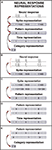Figure 1. Representations of the neural response. (A) In the spike representation, only the timing of action potentials is described, discarding the fine structure of the voltage traces. In the pattern representation, only the timing and categories of spike patterns remain. This representation is further transformed, to obtain the time and the category representations. The time (category) representation only keeps information about the timing (categories) of the spike patterns. (B), (C), (D), and (E) Each successive transformation of the neural response through a deterministic function simultaneously reduces both the variability in the neural response and number of possible responses.

From the spike representation, we can define more complex events, hereafter called patterns (see bold symbols in the spike representation in Figure 1A). Patterns may be defined in terms of spikes, bursts or ISIs (Alitto et al., 2005; Luna et al., 2005; Oswald et al., 2007; Eyherabide et al., 2008). They may involve one or several neurons. Examples of population patterns are coincident firing, precise firing events and sequences, or distributed patterns (Hopfield, 1995; Abeles and Gat, 2001; Reinagel and Reid, 2002; Gütig and Sompolinsky, 2006). The sequence of patterns obtained by transforming the spike representation is called the pattern representation. Analogously, the sequence of patterns only characterized by either their time positions or their categories constitute the time representation and category representation, respectively. Details on how to build these sequences are explained below. For simplicity, these sequences are represented in Figure 1 as sequences of symbols n, indicating specific events (n > 0) and silences (n = 0).

Formally, to obtain the spike representation (R), the unprocessed neural response (U) is transformed into a sequence of spikes (1) and silences (0) (Figure 1A). The time bin is taken small enough to include at most one spike. Differences in shape of action potentials are ignored, while their time positions are preserved, with temporal precision limited by the bin size. As a result, several sequences of action potentials may be represented by the same spike sequence (see Figure 1B).

In the pattern representation (B), the spike sequence is transformed into a sequence of silences (n = 0) and spike patterns (n = b > 0), distinguished solely by their category b. For example, in Figure 1, patterns are defined as response stretches containing consecutive spikes separated by at most one silence. The time positions of the patterns are defined as the first spike in each pattern stretch, whereas patterns with the same number of spikes are grouped into the same pattern category. Only information about pattern categories and time positions remains (compare the bold symbols in the spike and the pattern representation in Figure 1A). By ignoring differences among patterns within categories, several spike sequences can be mapped into the same pattern sequence, as shown in Figure 1C.

The time position of patterns is measured with respect to a common origin, in general, the beginning of the experiment. It can be defined, for example, as the first (or any other) spike of the pattern or as the mean response time (Lisman, 1997; Nelken et al., 2005; Eyherabide et al., 2009). Patterns are classified into categories according to different aspects describing their internal structure, such as the latency, the number of spikes or the spike-time dispersion (Theunissen and Miller, 1995; Gawne et al., 1996; Furukawa and Middlebrooks, 2002). Notice that latencies are usually defined with respect to the stimulus onset, which is not a response property (Chase and Young, 2007; Gollisch and Meister, 2008). Thus, latencies and timing of spike patterns are different concepts, and the latency cannot be read out from the neural response alone. However, latencies have also been defined with respect to the local field potential (Montemurro et al., 2008) or population activity (Chase and Young, 2007). These definitions can be regarded as internal aspects of spatiotemporal spike patterns (Theunissen and Miller, 1995; Nádasdy, 2000).

Categories of patterns can be built by discretizing the range of one or several internal aspects. For example, Reich et al. (2000) defined patterns as individual ISIs, and categorized them in terms of their duration. Three categories were considered, depending on whether the ISI was short, medium or large. In other cases, patterns may be sequences of spikes separated by less than a certain time interval. Categories of patterns can then be defined, depending on the number of spikes in each pattern (Reinagel and Reid, 2000; Martinez-Conde et al., 2002; Eyherabide and Samengo, 2010), as shown in Figure 1, or depending on the length of the first ISI (Oswald et al., 2007). The theory developed in this paper is valid irrespective of the way in which one chooses to define the pattern time positions and the pattern categories.

From the pattern sequence, we obtain the time representation (T) by only keeping the time positions of patterns. As a result, the neural response is transformed into a sequence of silences (0) and events (1), indicating the occurrence of a pattern in the corresponding time bin and disregarding its category. The temporal precision of the pattern representation is preserved in the time representation. However, by ignoring differences between categories, different pattern sequences can be mapped into the same time representation, as illustrated in Figure 1D.

The category representation (C) is complementary to the time representation. It is obtained from the pattern sequence, by only keeping information about the categories of patterns while ignoring their time positions. The neural response is transformed into a sequence of integer symbols n > 0, representing the sequence of pattern categories in the response. The exact time position of patterns is lost: only their order remains. Therefore, several pattern sequences may be mapped onto the same category sequence, as indicated in Figure 1E.

The spike (R), pattern (B), time (T), and category (C) representations are derived through functions that depend only on the previous representation, as denoted by the arrows in Figure 1A, and formally expressed by the following equations: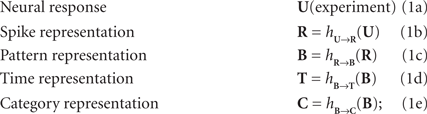where hXY represents the function h that is applied to the representation X to obtain the representation Y. These transformations progressively reduce both the variability in the neural response and the number of possible responses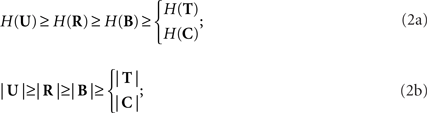where H(X) means the entropy H of the set X (Cover and Thomas, 1991), and |X| indicates its cardinality, i.e., the number of elements of the set X.

### 2.2 Calculation of Mutual Information Rates

The mutual information I(X; S) between two random variables X and S is defined as the reduction in the uncertainty of one of the random variables due to the knowledge of the other. It is formally expressed as a difference between two entropies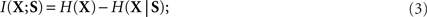where H(X) is the total entropy of X and H(X|S) represents the conditional or noise entropy of X provided that S is known (Cover and Thomas, 1991).

We estimate the mutual information between the stimulus S and a representation X of the neural response using the so-called Direct Method, introduced by Strong et al. (1998). The unprocessed neural response U is divided into time intervals Uτ of length τ. Each response stretch Uτ is then transformed into the discrete-time representation Xτ(Xτ = hUX(Uτ)), also called words. As a result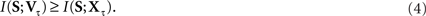This inequality is valid for every time interval of length τ (Cover and Thomas, 1991) and is not limited to the asymptotic regime for long time intervals, as in previous calculations (Gaudry and Reinagel, 2008; Eyherabide et al., 2009). The mutual information calculated with words of length τ only quantifies properly the contribution of spike patterns that are shorter than τ. In order to include the correlations between these patterns, even longer words are needed. Therefore, in this study, the maximum window length ranged between 3 and 4 times the maximum pattern duration.

The total entropy (H(Xτ)) and noise entropy (H(Xτ|S)) are estimated using the distributions of words Xτ unconditional (P(Xτ)) and conditional (P(Xτ|S)) on the stimulus S, respectively. The mutual information I(S; Xτ) is computed by subtracting H(Xτ|S) from H(Xτ) (Eq. 3). This calculation is repeated for increasing word lengths, and the mutual information rate I(S; X) between the stimulus S and a representation X of the neural response is estimated as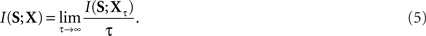This quantity represents the mutual information per unit time when the stimulus and the response are read out with very long words. In this work we always calculate mutual information rates unless it is otherwise indicated. However, for compactness, we sometimes refer to this quantity simply as “information.”

The estimation of information suffers from both bias and variance (Panzeri et al., 2007). In this work, the sampling bias of the information estimation was corrected using the NSB approach for the experimental data (Nemenman et al., 2004). For the simulations, we used instead the quadratic extrapolation (Strong et al., 1998), due to its simplicity and the possibility of generating large amounts of data. The standard deviation of the information was estimated from the linear extrapolation to infinitely long words (Rice, 1995). The bias correction was always lower than 1.5% and the standard deviation, always lower than 1%, for all simulations and all word lengths; thus error bars are not visible in the figures. When comparisons between information estimations were needed, one-sided t-tests were performed (Rice, 1995).

### 2.3 Simulated Data

Simulations are used to exemplify the theoretical results and to gain additional insight on how different response conditions affect information transmission in well-known neural models and neural codes. They represent highly idealized cases, with unrealistically long runs and number of trials, that allow us to readily exemplify the theoretical results and transparently obtain reliable information estimates. Firstly, we define the parameters used in the simulations and relate them to the specific aspects of the stimulus and the response. Then, we report the specific values for the parameters.

#### 2.3.1 General description

In the simulations, the stimulus consists of a random sequence of instantaneous discrete events, here called stimulus features. Each stimulus feature is characterized by specific physical properties, as for example, the color of a visual stimulus, the pitch of an auditory stimulus, the intensity of a tactile stimulus, or the odor of an olfactory stimulus (Poulos et al., 1984; Rolen and Caprio, 2007; Nelken, 2008; Mancuso et al., 2009). In the real world, however, features are not necessarily discrete. If they are continuous, one can discretize them by dividing their domain into discrete categories (Martinez-Conde et al., 2002; Eyherabide et al., 2008; Marsat et al., 2009). The present framework sets no upper limit to the number of features, nor to the similarity between different categories. In addition, features might not be instantaneous but rather develop in extended time windows, as it happens with the chirps in the weakly electric fish (Benda et al., 2005), the oscillations in the electric field potential (Oswald et al., 2007) and the amplitude of auditory stimuli (Eyherabide et al., 2008). In order to capture the duration of real stimuli, in the simulations we define a minimum inter-feature interval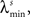for each feature s. After the presentation of a feature s, no other feature may appear in an interval lower or equal to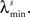In the simulated data, each stimulus feature elicits a neural response (see Figure 2A). Since in this paper we are interested in pattern-based codes, each feature generates a pattern of spikes belonging to some pattern category. The correspondence between stimulus features and pattern categories may be noisy. We consider both categorical noise (the pattern category varies from trial to trial) and temporal noise (the timing of the pattern varies from trial to trial). In Figure 2B, we show examples of all noise conditions using burst-like response patterns. In those examples, categories were defined according to the number of spikes in each burst.

FIGURE 2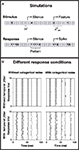Figure 2. Simulations: design and construction. (A) Example of a stimulus stretch and the elicited response. The stimulus is depicted as an integer sequence of silences (0) and features (s > 0), one symbol per time bin of size Δt. After a feature arrival, the stimulus remains silent for a periodThe response is represented as a binary sequence of spikes (1) and silences (0). Each stimulus feature elicits a response pattern: a burst containing n spikes. Different categories correspond to different intra-burst spike counts. (B) Examples of different response conditions. Upper panels: no temporal jitter; lower panels: the pattern, as a whole, is displaced due to temporal jitter; left panels: no categorical noise; right panels: each stimulus feature elicits pattern responses belonging to more than a single category.

Symbolically, the stimulus S is represented as a sequence of symbols s, one per time bin Δt. Each s is drawn randomly from the set of all possible outcomes Σs = {0, 1,…,NS}. The symbol s = 0 indicates a silence (the absence of a feature), whereas s > 0 tags the presence of a given feature. Each feature s elicits a response pattern r, drawn from the set Σr of all possible patterns, with probability Pr(r|s). The response pattern r may appear with latency μr, which might depend on the evoked pattern r. A neural response R, elicited by a sequence of stimulus features, may be composed of several response patterns (see bold symbol sequences in Figure 2A).

Figure 2B shows example neural codes with no noise (upper left panel), categorical noise alone (upper right), temporal noise alone (lower left), and a mixture of categorical and temporal noise (lower right). The categorical noise is defined by Pb(b|s), quantifying the probability that a response category b be elicited in response to stimulus s (see Appendix A for the relation between Pb(b|s) and Pr(r|s)). The temporal noise is implemented as jitter in the pattern onset time. That is, temporal jitter affects the pattern as a whole, displacing all spikes in the pattern by the same amount of time. The temporal displacement is drawn from a uniform distribution in the interval (−σb, σb), where the jitter σb may depend on the pattern b.

#### 2.3.2 Details and parameters

Simulated neural responses consisted of four different patterns, elicited by a stimulus with four different features. The response patterns were bursts of spikes, containing between 1 and 4 spikes. The intra-burst ISI was γmin = 2 ms. However, since the neural response is transformed into the pattern representation, the results are valid irrespective of the nature of the patterns (see Section 2.1). The stimulus was presented 200 times, each one lasting for 2000 s. The minimum inter-feature time interval is λmin = 12 ms. In all cases, no interference between patterns was considered (see Section 3.8). We used a time bin of size Δt = 1 ms.

Simulation 1. This simulation is used to illustrate the effect of using different representations of the neural response, and to compare an ideal situation where the correspondence between features and patterns is known, with a more realistic case, where the neural code is unknown. The temporal jitter was σ = 1 ms and the latency was μ = 1 ms. Stimulus features probability p(s) were set to: p(1) = 0.06, p(2) = 0.04, p(3) = 0.03, p(4) = 0.02. Categorical noise (p(b|s), b s): p(i + 1|i) = 0.1 (4 − i), 0 < i < 4; otherwise p(b|s) = 0.

Simulation 2. These simulations are used to address the role of the timing and category of patterns in the neural code, and to study the relation with the what and the when in the stimulus. The latency was μ = 1 ms. When present, temporal jitter was set to σ = 1 ms and categorical noise (p(b|s), b s) was given by: p(i + 1|i) = p(i|i + 1) = p(3|1) = p(2|4) = 0.1, 0 < i < 4; otherwise p(b|s) = 0. Stimulus features probability p(s) = 0.025, 0 < s ≤ 4.

### 2.4 Electrophysiology

Experimental neural data were provided by Ariel Rokem and Andreas V. M. Herz; they performed intracellular recordings in vivo, on the auditory nerve of Locusta Migratoria (see Rokem et al., 2006, for details). Auditory stimuli consisted of a 3 kHz carrier sine wave, amplitude modulated by a low pass filtered signal with a Gaussian distribution. The AM signal had a mean amplitude of 53.9 dB, a 6 dB standard deviation and a cut-off frequency of 25 Hz (see Figure 3A upper cell). Each stimulation lasted for 1000 ms with a pause of 700 ms between repeated presentations of the stimulus, in order to minimize the influence of slow adaptation. To eliminate fast adaptation effects, the first 200 ms of each trial were discarded. The recorded response (see Figure 3A lower panel) consisted of 479 trials, with a mean firing rate of 108 ± 6 spikes/s (mean ± standard deviation across trials). Burst activity was observed and associated with specific features in the stimulus (see Eyherabide et al., 2008, for the analysis of burst activity in the whole data set). Bursts contained up to 14 spikes; Figure 3B shows the firing probability distribution as a function of the intra-burst spike count.

FIGURE 3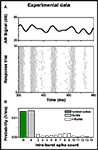Figure 3. Experimental data from a grasshopper auditory receptor neuron. (A) Upper panel: sample of the amplitude modulation of the sound stimulus used in the recordings. Lower panel: response to 30 of 479 repeated stimulus presentations showing conspicuous burst activity. Each vertical line represents a single spike. (B) Probability of firing a burst with n intra-burst spikes, in a time bin of size Δt = 1 ms. Isolated spikes (n = 1) and burst activity (n > 1) represent 49.4 and 50.6% of the firing events, respectively.

## 3 Results

### 3.1 Information Transmitted by Different Representations of the Neural Response: Spike and Pattern Information

In order to understand how stimuli are encoded in the neural response, the recorded neural activity U is transformed into several different representations. Each representation keeps some aspects of the original neural response while discarding others. The spike representation R is probably the most widely used (see Section 2.1). We define the spike information I(S; R) as the mutual information rate between the stimulus S and the spike representation R of the neural response.

The spike sequence can be further transformed into a sequence of patterns of spikes, called the pattern representation B. To that end, all possible patterns of spikes are classified into pre-defined categories, for example, burst codes, ISI codes, etc. (see Section 2.1 and references therein). We define pattern information I(S; B) as the information about the stimulus S, carried by the sequence of patterns B.

The pattern information cannot be greater than the spike information, which in turn cannot be greater than the information in the unprocessed neural response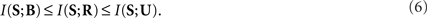This result can be directly proved from the deterministic relation between U, R and B (Eqs. 1) and the data processing inequality (Cover and Thomas, 1991). Notwithstanding, several neuroscience papers have reported data contradicting Eq. 6 (see Section 4.3). Intuitively, out of all the information carried by the unprocessed neural response, the spike information only contains the information preserved in the spike timing. Analogously, out of the information carried in the spike representation, the pattern information only preserves the information carried by both the time positions and the categories of the chosen patterns.

### 3.2 Choosing the Pattern Representation

In this paper, we quantify the amount of time and category information encoded by pattern-based codes. This information depends critically on the choice of the pattern representation. In this subsection, we discuss how to evaluate whether a given choice is convenient or not. One can choose any set of pattern categories to define the alphabet of the pattern representation. Some choices, however, preserve more information about the stimulus than others. The comparison between the information carried by different pattern representations gives insight on how relevant to information transmission the preserved structures are (Victor, 2002; Nelken and Chechik, 2007), i.e., formally, on whether they constitute sufficient statistics (Cover and Thomas, 1991). A suitable representation should reduce the variability in the neural response due to noise, while preserving the variability associated with variations in the encoded stimulus. Thus, any representation preserving less information than the spike information is neglecting informative variability. In addition, one may also be interested in a neural representation that can be easily or rapidly read out, or that is robust to environmental changes, etc. The chosen neural representation typically results from a trade-off between these requirements.

Here we focus on analyzing whether the chosen representation alters the correspondence between the stimulus and the response. For us, a good representation is one where the informative variability is preserved, and the non-informative variability is discarded. As an example, we analyze two different situations (Figure 4). In Figure 4A, we use simulated data, where we know exactly how the neural code is structured. We can therefore compare the performance of the spike representation, with two pattern representations: one of them intentionally tailored to capture the true neural code that generated the data, and another representation discarding some informative variability. The neural response consists of a sequence of four different patterns, associated with each of four stimulus features, in the presence of temporal jitter and categorical noise (see Section 2.3.2 Simulation 1). In Figure 4B, we study experimental data (see Section 2.4), so the neural code is unknown. Therefore, in this case we compare the spike representation with two candidate pattern representations, ignoring a priori which is the most suitable.

FIGURE 4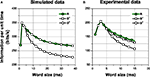Figure 4. Information per unit time transmitted by different choices of patterns. The spike representation (R) is transformed into a sequence of patterns grouped in categories according to the intra-pattern spike count (Bα), which is further transformed into a sequence of patterns classified as isolated spikes or complex patterns (Bβ). Comparing the amount of information transmitted gives insight about the relevance of the structures preserved in the representations. (A) Simulation of a neural response with four different patterns, elicited by a stimulus with four different features, in presence of temporal jitter and categorical noise (see Section 2.3.2 Simulation 1 for details). (B) Experimental data from a grasshopper auditory receptor neuron. In all cases, error bars <1% (smaller than the size of the data points).

For both simulation and experimental data, we estimated the information conveyed by the spike representation R; a pattern representation Bα, where all bursts are grouped into categories according to their intra-burst spike count; and a second pattern representation Bβ, with only two categories comprising isolated spikes and complex patterns. This is shown in Figure 4, where the information per unit time is plotted as a function of the window size used to read the neural response. The representations are related through functions, in such a way that Bβ is a transformation of Bα, which is in turn a transformation of R. Therefore, I(S; Bβ) ≤ I(S; Bα) ≤ I(S; R), for all finite response windows (see Eq. 6). Nevertheless, notice that Bβ may be a faster-to-read code than Bα, since the latter requires a time window long enough to distinguish not only the differences between isolated spikes and bursts, but also the differences among bursts of different categories.

In the simulation (Figure 4A), the information carried by Bα is equal to the spike information (ISim(S; R) = ISim(S; Bα) = 254.2 ± 0.2 bits/s, one-sided t-test, p(10) = 0.5). This is expected since, by construction, the neural code used in the simulations is, indeed, Bα. Therefore, in this case, Bα is a lossless representation. The choice of an adequate representation is more difficult in the experimental example (Figure 4B), where the neural code is not known beforehand. In this case, Bα preserves less information than the spike sequence (IExp(S; R) = 133 ± 4 bits/s, IExp(S; Bα) = 121 ± 3 bits/s, one-sided t-test, p(10) = 0.004). The information I(S; Bα) represents 91% of the spike information. In general, whether this amount of information is acceptable or not depends on whether the loss is compensated by the advantages of attaining a reduced representation of the response (Nelken and Chechik, 2007).

Distinguishing only between isolated spikes and bursts (Bβ) diminishes the information considerably in both examples (one-sided t-test, p(10) < 0.001, both cases). In the simulation, the information carried by Bβ is ISim(S; Bβ) = 208.7 ± 0.6 bits/s, representing about 82.1% of the spike information. This is expected since, by construction, different stimulus features are encoded by different patterns. For the experimental data, IExp(S; Bβ) = 91 ± 7 bits/s, representing about 68% of the spike information. In both examples, the representation Bα is “more sufficient” than Bβ. The difference I(S; Bα) − I(S; Bβ) constitutes a quantitative measure of the role of distinguishing between bursts of 2, 3, …, n spikes, provided that the distinction between isolated spikes and bursts has already been made (I(S; Bα|Bβ). However, Bα still preserves other response aspects, such as pattern timing, number of patterns, etc. In what follows, we study the role of different response aspects in information transmission.

### 3.3 Informative Aspects of the Neural Response

The pattern representation may preserve one or several aspects of the neural response that could, in principle, encode information about the stimulus. More specifically, if the response is analyzed using windows of duration τ, there are several candidate response aspects that might be informative, namely:

1. the number of patterns in the window (number of events – Figure 5A)

2. the precise timing of each pattern in the window (time representation – Figure 1D)

3. the pattern categories present in the window with no specification of their ordering (response set of categories – Figure 5B)

4. the temporally ordered pattern categories in the window (category representation – Figure 1E).

FIGURE 5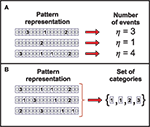Figure 5. Identifying the information carriers in the neural response. (A) The representation η of the neural response is obtained by transforming the pattern sequence such that only the number of events is preserved. (B) By transforming the pattern sequence into the representation Θ, the information about the categories present in the neural response is preserved, while their order of occurrence is disregarded.

We find that these aspects are related through deterministic functions. Indeed, aspect a can be univocally determined from aspects b, c or d. Thus, the information transmitted by aspect a is also carried by any of the other aspects. In the same manner, aspect c can be determined from d. However, in Appendix B we prove that the number of patterns in the window (aspect a) makes a vanishing contribution to the information rate. That is, although aspect a might be informative for a finite window of length τ, its contribution becomes negligible in the limit of long windows. Surprisingly, the unordered set of pattern categories (aspect c) also makes no contribution to the information rate, as shown in Appendix C. Even more, the entropy rates of both aspects tend to zero in the limit of long time windows. Therefore, their information rate with respect to any other aspect, of either the stimulus and/or the neural response, vanishes as the window size increases. We thus do not discuss aspects a and c any further.

This is not the case of response aspects b and d. In other words, they may sometimes be informative; their definitions do not constrain them to be non-informative. Therefore, in what follows, we transform the pattern representation into two other representations preserving the precise timing of each pattern (the time representation) and the temporally ordered pattern categories (the category representation). Our goal is to determine in which way the precise timing of each pattern conveys information about the time positions of stimulus features (the when), and how the temporally ordered pattern categories provide information about the identity of the stimulus features (the what).

### 3.4 Time and Category Information

We define the time information I(S; T) as the mutual information rate between the stimulus S and the time representation T. In addition, we define the category information I(S; C) as the mutual information rate between the stimulus S and the category representation C. The category information is novel and, unlike and complementing previous studies (Gaudry and Reinagel, 2008; Eyherabide et al., 2009), allows us to address the relevance of pattern categories in the neural code (see Section 3.5). Since both T and C are transformations of the pattern representation B (see Eqs 1), the time and category information cannot be greater than the pattern information, i.e.,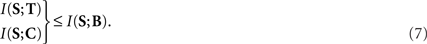When T and C are read out simultaneously, the pair (T,C) carries the same information as the pattern sequence B (I(T,C; S) = I(B; S)). In fact, B and the pair (T,C) are related through a bijective function. To prove this, consider any pattern representation Bi of a neural response Ui. The pair (Ti, Ci) associated with Ui is a function of Bi (see Eqs. 1). Conversely, given the pair (Ti, Ci) associated with Ui, all the information about the time positions and categories of patterns present in Ui is available, and thus Bi is univocally determined. Notice that the pairs (T, C) are a subset of the Cartesian product T × C.

The time positions of patterns may depend on their categories, and vice versa. To explore this relationship, and how it affects the transmitted information, we separate the pattern information as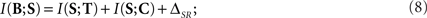where ΔSR represents the synergy/redundancy between the time and the category representations, defined by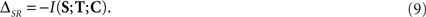Here, I(X; Y; Z) = I(X; Y) − I(X; Y|Z) is called triple mutual information (Cover and Thomas, 1991; Tsujishita, 1995). If ΔSR is positive, time and category information are synergistic: more information is available when T and C are read out simultaneously. Conversely, if ΔSR is negative, time and category information are redundant. The proof of Eqs. 8 and 9 is shown in Appendix D. Previous studies have already defined the synergy/redundancy for populations of neurons (Schneidman et al., 2003). It has also been applied to single neurons, to determine how different aspects of response patterns encode the identity of single stimulus features (Furukawa and Middlebrooks, 2002; Nelken et al., 2005). Here we extend the concept to encompass also dynamic stimuli where stimulus features arrive at random times, as well as for arbitrary patterns, defined in time and/or across neurons.

As an example, consider the data presented in Figure 4, when the neural responses represented as a sequence of bursts (Bα). For the case of the simulations (Figure 4A), the time information is ISim(S, Tα) = 180.4 ± 0.2 bits/s, and the category information, ISim(S, Cα) = 74.2 ± 0.5 bits/s. The synergy/redundancy term is slightly negative, but not significant (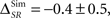two-sided t-test, p(15) = 0.44). By construction, in the simulation the time and category information are neither redundant nor synergistic. For the experimental data (Figure 4B), IExp(S, Tα) = 63 ± 2 bits/s and IExp(S, Cα) = 50.6 ± 0.6 bits/s. In this case, we do not know whether the time information and the category information are redundant or synergistic beforehand. Yet, by comparing them with the pattern information we obtain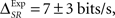indicating that timings and categories of patterns are slightly synergistic (two-sided t-test, p(15) = 0.063).

The pattern, time and category information depend on the choice of the alphabet of patterns. For example, the category information may increase or decrease depending on the nature of the aspect defining the pattern categories (Furukawa and Middlebrooks, 2002; Gollisch and Meister, 2008). No general rules can be given, predicting these changes: they depend on the neural representation at hand. However, when the alternative pattern representations are linked through functions, some relations between their variations can be predicted, without numerical calculations. Compare, for instance, Bα and Bβ as defined in Section 3.1. By grouping all bursts with more than one spike into a single category, not only Bβ is a function hα→β of Bα (Bβ = hα→β(Bα)), but also Cβ = hα→β(Cα). The time representation remains intact (Tβ = Tα). As a result, neither the pattern information nor the category information can increase, whereas the time information remains constant. In addition, if Tα and Cα are independent and conditionally independent given the stimulus, so are Tβ and Cβ. Therefore, the difference in the category information equals the difference in the pattern information (I(S; Cα) − I(S; Cβ) = I(S; Bα) − I(S; Bβ)).

Analogously, consider a representation Bγ in which the time positions of patterns identified in Bα are read out with lower precision (2 Δt). Since Bγ is a function of Bα, two different responses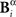and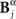that only differ little in the pattern time positions are indistinguishable in the representation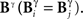In this case, the comparison between Bα and Bγ is analogous to the case analyzed in the previous paragraph, with the role of the time and category representations interchanged.

We illustrate these results with an example. In Figure 6, the pattern, time and category information are shown for three different choices of the pattern representation. The simulated neural response is taken from Figure 4A. In the three cases, there is no synergy or redundancy between the time and the category information (ΔSR = 0). From Figure 4A, we already know that I(S; Bβ) < I(S; Bα). Comparing the left and middle panels of Figure 6, we find that this reduction is due to a decrement in the category information (I(S, Cα) = 74.2 ± 0.5 bits/s, I(S, Cβ) = 28.6 ± 0.3 bits/s, one-sided t-test, p(10) < 0.001), as expected (see Section 3.1). In agreement with the theoretical prediction, the time information remains unchanged (I(S, Tα) = I(S, Tβ) = 180.4 ± 0.2 bits/s, one-sided t-test, p(10) = 0.5).

FIGURE 6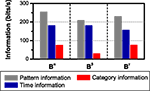Figure 6. Pattern, time and category information carried by different neural representations. The spike representation is transformed into a sequence of patterns: Bα (left): grouped in categories according to the intra-pattern spike count; Bβ (middle): classified as isolated spikes or complex patterns; and Bγ (right): classified as in Bα, reading out the time positions with a lower precision (2 Δt). In all cases, error bars <1%. The simulation data is taken from Figure 4 (see Section 2.3.2 Simulation 1 for details).

Analogously, compare the left and right panels of Figure 6. In this case, both the pattern and time information decrease (I(S, Bα) = 254.2 ± 0.2 bits/s, I(S, Bγ) = 230.1 ± 0.1 bits/s, I(S, Tα) = 180.4 ± 0.2 bits/s, I(S, Tγ) = 156.0 ± 0.2 bits/s, in both cases, one-sided t-test, p(10) < 0.001), while the category information remains unchanged (I(S, Cα) = I(S, Cγ) = 74.2 ± 0.5 bits/s, one-sided t-test, p(10) = 0.5). Thus, as mentioned previously, a reduction in the precision with which the patterns are read out always decreases the time information, while keeping the category information constant.

In other examples, the variations in the time and category information may not be directly accompanied by variations in the pattern information, due to the presence of synergy and redundancy. For example, Alitto et al. (2005) studied the encoding properties of tonic spikes, long-ISI tonic spikes (tonic spikes preceded by long ISIs) and bursts. To evaluate the relevance of distinguishing between tonic spikes and long-ISI tonic spikes, one can compare the information conveyed by two representations: Bξ, preserving the difference between tonic spikes and long-ISI tonic spikes, and Bφ, grouping them into the same category (Gaudry and Reinagel, 2008). Both Bξ and Bφ only differ in the category representation, like Bα and Bβ. However, unlike those representations,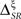and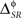need not be either equal or zero, and thus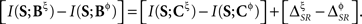Indeed, by reading simultaneously the timing and category of a pattern, the uncertainty on whether the following pattern will be a long-ISI tonic spike is reduced. Hence, this reduction is a source of redundancy in Bξ, where the long-ISI tonic spikes are explicitly identified. On the other hand, the inter-pattern time interval (IPI) preceding a long-ISI tonic spike may reveal the duration of the previous pattern. Any information contained in it constitutes a source of synergy in Bξ. The distinction between tonic spikes and bursts produces analogous effects on the synergy and redundancy, affecting both representations Bξ and Bφ.

As shown in Cover and Thomas (1991), I(S; T; C) is symmetric in S, T and C. Hence, ΔSR is upper and lower-bounded by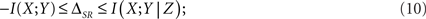where X, Y and Z represent the variables S, T and C in such an ordering that I(X; Y) = min{I(T; C),I(S; T),I(S; C)} (see proof in Appendix E). The same ordering applies for both bounds, in such a way that, for example, if I(S; T|C) is the least upper-bound, then I(S; T) is the greatest lower-bound, from the set of bounds derived in Eq. 10. These bounds are novel, tighter than the bounds previously mentioned Schneidman et al. (2003).

If the left side of Eq. 10 is zero, time and category information are non-redundant (ΔSR ≥ 0). However, they may still be synergistic (0 ≤ ΔSR), even in the case when they are both zero (I(S; T) = I(S; C) = 0 ⇒ ΔSR ≥ 0). This property has often been overlooked (see, for example, Foffani et al., 2009). Time and category information are non-synergistic if and only if the right side of Eq. 10 is zero. From the definition of the synergy/redundancy ΔSR (Eq. 9), we show that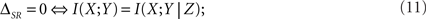where X, Y and Z represent the variables S, T and C in any order. In this case, the time and category information add up to the pattern information. This situation may occur when either I(X; Y) = I(X; Y|Z) = 0 or I(X; Y) = I(X; Y|Z) > 0 (Nirenberg and Latham, 2003; Schneidman et al., 2003).

### 3.5 Relevance and Sufficiency of Different Aspects of the Neural Response

Previous studies have addressed the relevance of pattern timing in information transmission by quantifying the time information and comparing it with the pattern information (Denning and Reinagel, 2005; Gaudry and Reinagel, 2008; Eyherabide et al., 2009). In other words, the relevance of pattern timing is given by the amount of information carried by a representation that only preserves the time positions of patterns. We call this paradigm criterion I. Indeed, one can also address the relevance of pattern categories using criterion I. However, instead of quantifying the amount of information carried by the category representation, these previous works have determined the information loss due to ignoring the pattern categories. Here, this point of view is called criterion II. In what follows, we prove that criterion I and criterion II take into account different information, and can thus lead to opposite results when both of them are applied to the same aspect of the response.

Formally, under criterion I, the pattern timing is relevant (or sufficient) for information transmission if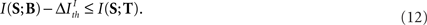Here,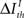represents a previously set threshold. Although Cover and Thomas (1991) have defined sufficiency only for the case when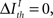in practice, some amount of information loss (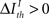) is usually accepted (Nelken and Chechik, 2007). We can also employ this criterion to address the relevance of pattern categories, comparing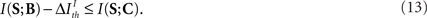On the other hand, under criterion II, the pattern categories are relevant to information transmission if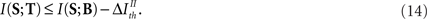Therefore, pattern categories are relevant if pattern timings transmit little information, irrespective of the information carried by categories themselves. Remarkably, if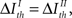the pattern categories are relevant (irrelevant) if and only if the pattern timings are irrelevant (relevant) (compare Eqs. 12 and 14).

From the bijectivity between B and (T; C) (see Section 3.4), we find that criterion II can be written as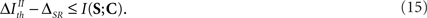As a result, under criterion II, the relevance of an aspect depends not only on the information conveyed by that very aspect – as in criterion I – but also on the synergy/redundancy between that aspect and the complementary ones. Both criteria coincide when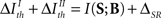(compare Eqs. 13 and 15), implying that equality in the thresholds is neither necessary nor sufficient to obtain a coincidence.

By using criterion I for the relevance of pattern timing and criterion II for the relevance of pattern categories, the information that is repeated in both aspects (redundant information) only contributes to the relevance of the pattern timing. However, the information that is carried in both aspects simultaneously (synergistic information) only contributes to the relevance of the pattern categories. The discrepancies in this way induced are shown in the following example. Consider that I(S; R) = 10 bits/s, I(S; T) = 9 bits/s, I(S; C) = 10 bits/s and ΔIth = 2 bits/s. Under criterion II, C is irrelevant because I(S; B) − I(S; T) = 1 bit/s. Nevertheless, under criterion I, C is necessarily relevant, since it constitutes a sufficient statistics (I(S; B) = I(S; C)). Analogous results are obtained for the relevance of pattern timing. In addition, different thresholds are used for the relevance of each aspect (compare Eqs. 12 and 14). In the previous example, the pattern timing is relevant only if I(S; T) > 8 bits/s whereas the pattern categories are relevant only if I(S; C) > 2 bits/s, showing an unjustified asymmetry between both aspects.

### 3.6 Time and Category Entropy of the Stimulus

Many studies have interpreted that pattern-based codes function as feature extractors, where the identity of each stimulus feature (the what) is represented in the pattern category C, and the timing of each stimulus feature (the when), in the pattern temporal reference T (see Introduction and references therein). To assess this standard view, we formally define the what and the when in the stimulus, and relate them with the time and category information. In the next subsection, we determine the conditions that are necessary and sufficient for the standard view to hold. Finally, we show that small category-dependent changes in the timing of patterns (such as latencies) may induce departures from the standard view (altering both the amount and the composition of the information carried by T and C).

Since the stimulus S is composed of discrete features (see Methods for a discussion on continuous stimuli), it can also be written in terms of a time (ST) and a category (SC) representation, such that S and the pair (ST, SC) are related through a bijective map. We formally define the what in the stimulus as the category representation SC, and the when as the time representation ST. Indeed, ST indicates when the stimulus features occurred, whereas SC tags what features appeared.

The stimulus entropy is defined as the entropy rate H(S), while the stimulus time entropy and category entropy are the entropy rates H(ST) and H(SC), respectively. The time and category entropies are intimately related to when and what features happened: they are a measure of the variability in the time positions and categories of stimulus features, respectively. These quantities were previously defined for Poisson stimuli in Eyherabide and Samengo (2010), and here these definitions are generalized to encompass any stochastic stimulus. Since S and (ST, SC) are related through a bijective function,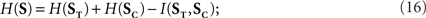where the information rate I(ST, SC) is a measure of the redundancy between the time and category entropies of the stimulus. Since I(ST, SC) is always non-negative, ST and SC cannot be synergistic.

The standard view of the role of patterns formally implies that the category information I(S, C) (the time information I(S, T)) can be reduced to the mutual information I(SC, C)(I(ST, T)). Therefore, H(SC) and H(ST) must be upper-bounds for the category and time information, respectively. However, these bounds are not guaranteed by the mere presence of patterns in the neural response. Some cases may be more complicated because, for example, SC and ST may not be independent variables (see Section 2.3). A dependency between these two stimulus properties implies that the what and the when are not separable concepts.

### 3.7 The Canonical Feature Extractor

In this section, we determine the conditions under which the standard interpretation holds: The category information represents the knowledge on the what in the stimulus, and the time information, the knowledge on the when. To that aim, we define a canonical feature extractor as a neuron model in which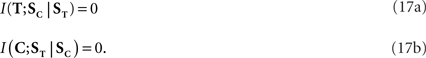Under each of these conditions, the time and category information become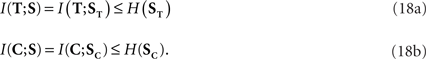Consequently, the response pattern categories represent what stimulus features are encoded, whereas the pattern time positions represent when the stimulus features occur. In particular, the time and category information are upper-bounded by the stimulus time and category entropies, respectively.

Condition 17a implies that all the information I(SC; T) is already contained in the information I(ST; T). In other words, I(SC; T) is completely redundant with I(ST; T), and I(SC; T) ≤ I(ST; T). In this sense, we say that the time information represents the when in the stimulus. Analogous implications can be obtained from condition 17b for the category representation C, by interchanging T with C, and ST with SC (see formal proof in Appendix F). Therefore, conditions 17 are necessary and sufficient to ensure that the standard view of the role of patterns in the neural code actually holds (see Section 4.1).

A canonical feature extractor does not require T and C to be independent nor conditionally independent given the stimulus. In other words, the time and category information may or may not be synergistic or redundant, and the timing (category) of each individual pattern may or may not be correlated with other pattern time positions (pattern categories) or even with pattern categories (pattern time positions). In addition, conditions 17 may also encompass situations in which some information about SC (ST) is carried by T (C), but not by C (T).

In order to see how synergy and redundancy behave in a canonical feature extractor, we replace Eqs. 18 in Eq. 8, and obtain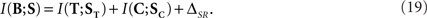We find that, for a canonical feature extractor, the synergy/redundancy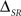is lower-bounded by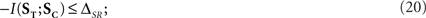(see proof in Appendix G). In other words, the synergy/redundancy term ΔSR cannot be smaller than the redundancy – already present in the stimulus – between the timing and categories of stimulus features. In addition, the absence of redundancy in the stimulus (I(ST; SC) = 0) constrains the neural model to be non-redundant (ΔSR ≥ 0).

Consider a neural model in which T = f(STT) and T = f(SCC), where ψT and ψC are independent sources of noise, such that pT, ψC, ST, SC) = pT) pC) p(ST, SC). Thus, T and C are two channels of information under independent noise (Shannon, 1948; Cover and Thomas, 1991). This model constitutes a canonical feature extractor. Indeed, T (C) is only related to SC (ST) through ST (SC), thus complying with condition 17a (17b). In addition, if ST and SC are independent, then T and C constitute independent channels of information (Cover and Thomas, 1991; Gawne and Richmond, 1993). This model plays a prominent role in the interpretation of neurons and neural pathways as channels of information (Gawne and Richmond, 1993; Schneidman et al., 2003; Montemurro et al., 2008; Krieghoff et al., 2009), as discussed in Section 4.5.

The independent channels of information may be regarded as the simplest canonical feature extractor. Since T and C are independent and conditionally independent given S, the time and category information add up to the pattern information (ΔSR = 0). An example of this model is shown in Figure 7. In the four simulations carried out, the neural responses consist of a sequence of four different patterns, associated with four different stimulus features, under the presence or absence of temporal jitter and categorical noise (see Section 2.3.2 Simulation 2 for a detailed description; Figure 2B shows examples of the different noise conditions). In Figure 7, the spike information is omitted because it coincides with the pattern information (all cases, one-sided t-test, p(10) = 0.5). Indeed, by construction, all the information is transmitted by patterns, which can be univocally identified in the response. In agreement with the theoretical results (Eq. 18), the time and the category information are always upper-bounded by the stimulus time and category entropy, respectively (all cases, one-sided t-test, p(10) > 0.4).

FIGURE 7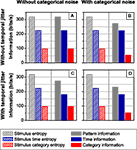Figure 7. Information transmitted by a canonical feature extractor under different noise conditions. The left side of each panel shows the stimulus entropy, whereas the right side shows the pattern, time and category information. In all cases, ΔSR = 0, so the pattern information is equal to the sum of the category and the time information. From left to right: Absence (A,C) and presence (B,D) of categorical noise. The addition of categorical noise reduces only the category information irrespective of the amount of temporal jitter. From top to bottom: Absence (A,B) and presence (C,D) of temporal jitter. The presence of temporal jitter degrades solely the time information irrespective of the amount of categorical noise. The pattern information is upper bounded by the stimulus entropy, the time information by the stimulus time entropy, and the category information by the stimulus category entropy. In all cases, error bars <1%. For detailed description of the simulation see Section 2.3.2 Simulation 2.

Comparing upper and lower panels of Figure 7, we show that the time information is degraded by the addition of temporal jitter (both cases, one-sided t-test, p(10) < 0.001), while the category information remains constant (both cases, one-sided t-test, p(10) > 0.14). Analogously, comparing left and right panels of Figure 7, we find that the addition of categorical noise decreases the category information (both cases, one-sided t-test, p(10) < 0.001), while keeping the time information constant (Figures 7A,B, I(S; TA) = 223.3 ± 0.1 bits/s, I(S; TB) = 222.8 ± 0.1 bits/s, one-sided t-test, p(10) = 0.08; Figures 7C,D, one-sided t-test, p(10) = 0.5). This is expected since, by construction, the categorical noise only depends on the stimulus categories and affects solely the pattern categories, whereas the temporal jitter considered here only affects the pattern time positions, irrespective of their categories or the stimulus.

### 3.8 Departures From the Canonical Feature Extractor

The example shown in Figure 7 turns out to be more complicated if the pattern timing depends on the pattern category, as occurs in latency codes (Gawne et al., 1996; Furukawa and Middlebrooks, 2002; Chase and Young, 2007; Gollisch and Meister, 2008). Indeed, in those cases, the comparison between the timing of response patterns and the timing of stimulus features carries information about the stimulus categories (I(SC; T|ST) > 0). As a result, Eq. 17a does not hold. Latency codes may be an intrinsic property of the encoding neuron, may result as a consequence of synaptic transmission (Lisman, 1997; Reinagel et al., 1999), or may either arise from the convention used to construct the pattern representation, for example, ascribing the timing of a pattern as the mean response time, the first or any other spike inside the pattern (Nelken et al., 2005; Eyherabide et al., 2008). In all these cases, a latency-like dependence between the time positions and categories of patterns may arise.

To assess the effect of different latencies associated with each pattern category on the neural response, consider the neural model used in Figure 7, except that now, the pattern latencies vary with the pattern category b, according to μb = 1 + αμ*(4 − b). Here αμ is the latency index, representing the difference between the latencies of consecutive pattern categories. Three values of αμ were considered: 0, 2, and 4 ms. When αμ = 0 ms, all patterns have the same latencies. This case was analyzed in Figure 7. As αμ increases, so does the latency difference of different patterns.

Due to the deterministic link between the pattern latencies and pattern categories, the pattern representations (B0, B2, and B4), associated with the different values of αμ are related bijectively. In addition, the category representation does not depend on αμ. Only the time representation is altered by a change in the latency index, irrespective of the presence of absence of temporal jitter and categorical noise. Therefore, any change in the time information is immediately reflected in the synergy/redundancy term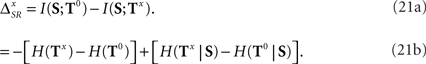Here,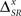and Tx represent the synergy/redundancy term and the time representation, respectively, for αμ = x ms.

The impact of different latencies is twofold. In the first place, the presence of categorical noise increments the temporal noise through the deterministic link between latencies and categories. Therefore, the time noise entropy (time information) when αμ > 0 is greater (less) than that when αμ = 0. However, this does not occur when the time and category representations are read out simultaneously. Indeed, given the category representation, any time representation for αμ = x > 0 can be univocally determined from the time representation for αμ = 0, and vice versa, counteracting the effect of the temporal noise. Therefore, the variation in the time noise entropy (H(Tx|S) − H(T0|S) in Eq. 21) can be regarded as a source of synergy.

In the second place, the variation in the latencies modifies the inter-pattern time interval distribution, incrementing the time total entropy (and the time information) when αμ > 0 with respect to the case when αμ = 0. In addition, this variation introduces information about the pattern categories in the inter-pattern time interval, and consequently it also introduces information about the stimulus identities. For example, a short interval between two consecutive patterns indicates that the second patterns belongs to a category with a short latency. In consequence, the increment in the time total entropy (H(Tx) − H(T0) in Eq. 21) can be regarded as a source of redundancy.

To illustrate these theoretical inferences, the results of the simulations are shown in Figure 8. As expected, when αμ = x > 0, the latencies alter the time information. However, they do not alter the pattern nor the category information, and thus any variation in the time information is compensated by an opposite variation in the synergy/redundancy term. Notice that the changes in the time information not only depend on the latency index, but also on the presence of temporal and categorical noise. Indeed, in the absence of categorical noise, (H(Tx|S) = H(T0|S) = 0, and thus ΔSR ≤ 0. The effect of the temporal jitter depends on its distribution as well as the distribution of the inter-pattern time intervals, so this analysis if left for future work.

FIGURE 8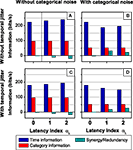Figure 8. Examples of departures from the behavior of the canonical feature extractor: The effect of pattern-category dependent latencies. From left to right: Absence (A,C) and presence (B,D) of categorical noise. From top to bottom: Absence (A,B) and presence (C,D) of temporal jitter. In all cases, when latencies depend on the pattern category, the time information is affected while the category information remains unchanged. Furthermore, the addition of categorical noise not only affects the category information but also the time information. In general, how the addition of temporal and/or categorical noise affects the time information depends on the latency index, as well as on the noise already present in the response. For simulation details, see Section 2.3.2 Simulation 2. The case where αμ = 0 ms was analyzed in Figure 7 and is reproduced here for comparison. In (A), the case where αμ = 0 ms also represents the stimulus entropies, as shown in Figure 7A. In all cases, error bars <1%.

In these examples we see that for non-canonical feature extractors, one can no longer say that the pattern categories represent the what in the stimulus and the pattern timings represent the when, not even in the absence of synergy/redundancy. As shown in Eq. 21, ΔSR results from a complex trade-off between the effect of categorical noise on the total and noise time response entropies. This trade-off depends on the latency index and the amount of temporal noise in the system, as shown in Figure 8.

Latency-like effects may be involved in a translation from a pattern duration code into an inter-spike interval code (Reich et al., 2000; Denning and Reinagel, 2005). Indeed, bursts may increase the reliability of synaptic transmission (Lisman, 1997), making it more probable to occur at the end of the burst. In that case, the duration of the burst determines the latency of the postsynaptic firing. In particular, this indicates that bursts can be simultaneously involved in noise filtering and stimulus encoding, in spite of the belief that these two functions cannot coexist (Krahe and Gabbiani, 2004). Notice that here, latency codes have been studied for well-separated stimuli. However, if patterns are elicited close enough in time, they may interfere in a diversity of manners (Fellous et al., 2004), precluding the code from being read out. Although we cannot address all these cases in all generality, the framework proposed here is valid to address each particular case.

## 4 Discussion

In this paper, we have focused on the analysis of temporal and categorical aspects, both in the stimulus and the response. Our results, however, are also applicable to other aspects. In the case of responses, these aspects can be latencies, spike counts, spike-timing variability, autocorrelations, etc. Examples of stimulus aspects are color, contrast, orientation, shape, pitch, position, etc. The only requirement is that the considered aspects be obtained as transformations of the original representation, as defined in Section 2.1 (see Section 4.2). The information transmitted by generic aspects can be analyzed by replacing B (S) with a vector representing the selected response (stimulus) aspects. The amount of synergy/redundancy between aspects is obtained from the comparison between the simultaneous and individual readings of the aspects. In addition, the results can be generalized for aspects defined as statistical (that is, non-deterministic) transformations of the neural response, or of the stimulus. The data processing inequality also holds in those cases (Cover and Thomas, 1991).

### 4.1 Meaning of Time and Category Information and their Relation with the what and the when in the Stimulus

In this paper, we defined the category and the time information in terms of properties of the neural response. The category (time) information is the mutual information between the whole stimulus S and the categories C (timing T) of response patterns (see Figure 9A). These definitions only require the neural response to be structured in patterns. No requirement is imposed on the stimulus, i.e., the stimulus need not be divided into features. Our definitions, hence, are not symmetric in the stimulus and the response. In some cases, however, the stimulus is indeed structured as a sequence of features. One may ask how the stimulus identity (the what) and timing (the when) is encoded in the neural response (see Figure 9B). To that end, we defined the what in the stimulus in terms of the category representation (SC), and the when, in terms of the time representation (ST).

FIGURE 9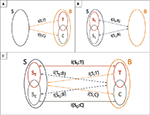Figure 9. Analysis of the role of spike patterns: relationship with the what and the when in the stimulus. (A) Categorical and temporal aspects in the neural response. Definitions of time I(S; T) and category I(S; C) information. (B) Categorical and temporal aspects in the stimulus. Information about the what I(SC; B) and the when I(ST; B) conveyed by the neural response B. (C) Analysis of the role of patterns in the neural response. Mutual information between different aspects of the stimulus and different aspects of the neural response.

These rigorous definitions allowed us to disentangle how the what and the when in the stimulus are encoded in the category and time representations of the neural response. We calculated the mutual information rates between different aspects of the stimulus and different aspects of the neural response (see Figure 9C). In the standard view, the pattern categories are assumed to encode the what in the stimulus, and the timing of patterns, the when (Theunissen and Miller, 1995; Borst and Theunissen, 1999; Martinez-Conde et al., 2002; Krahe and Gabbiani, 2004; Alitto et al., 2005; Oswald et al., 2007; Eyherabide et al., 2008). These assumptions have been stated in qualitative terms. There are two different ways in which the standard view can be formalized as a precise assertion.

On one hand, the standard view can be seen as the assumption that the category (time) representation only conveys information about the what (the when). Evaluating this assumption involves the comparison between the information conveyed by the category (time) representation about the whole stimulus (dotted lines in Figure 9C) with the information that this same representation conveys about the what (the when) in the stimulus (solid lines in Figure 9C). Formally, this means to address whether I(S; C) = I(SC; C) (whether I(S; T) = I(ST; T)). In this sense, we say that the category (time) information only represents the what (the when) in the stimulus. A system complying with this first interpretation of the standard view was called a canonical feature extractor (see Section 3.7).

On the other hand, the second way to define the standard view rigorously is to assume that the what (the when) is completely encoded by the category (time) representation. Testing this second assumption involves the comparison between the information about the what (the when), conveyed by the category (time) representation (solid lines in Figure 9C) and by the pattern representation of the neural response (dashed lines in Figure 9C). Formally, it involves assessing whether I(SC; B) = I(SC; C) (whether I(ST; B) = I(ST; T)). In this sense, we say that all the information about the what (the when) in the stimulus is encoded in the category (time) representation of the neural response. A system for which these equalities hold is called a canonical feature interpreter. It is analogous to the canonical feature extractor, with the role of the stimulus and the response interchanged (see Appendix H).

The two formalizations of the standard view are complementary. The first one assesses how different aspects of the stimulus are encoded in each aspect on the neural response. The second one focuses on how each aspect of the stimulus is encoded in different aspects of the neural response. Thus, the second approach is a symmetric version of the first one. However, a canonical feature extractor might or might not be a canonical feature interpreter, and vice versa. A perfect correspondence between the what and the when on one side, and pattern timing and categories, on the other, is found for systems that are canonical feature extractors and canonical feature interpreters, simultaneously.

### 4.2 Two Different Approaches to the Analysis of Neural Codes

In order to understand a neural code, one needs to identify those aspects of the neural response that are relevant to information transmission. To that aim, two different paradigms have been used: criterion I, assessing the information that one aspect conveys about the stimulus, and criterion II, assessing the information loss due to ignoring that aspect (see Section 3.5). Previous studies have used criterion I to analyze the relevance of spike counts (Furukawa and Middlebrooks, 2002; Foffani et al., 2009), spike patterns (Reinagel et al., 1999; Eyherabide et al., 2008), and pattern timing (Denning and Reinagel, 2005; Gaudry and Reinagel, 2008; Eyherabide et al., 2009). However, when assessing the relevance of the complementary aspects, such as spike timing and internal structure of patterns, these studies have used criterion II. As a result, in these studies the relevance of the tested aspect is conditioned to the irrelevance of the other aspects.

There are cases where building a representation that preserves a definite response aspect is not evident (nor perhaps possible). Such is the case, for example, when assessing the differential roles of spike timing and spike count: It is not possible to build a representation preserving the timing of the spikes without preserving the spike count (see Section 3.3). It is instead possible to only preserve the spike count. Since the spike-count representation is a function of the spike-timing representation, one may argue that there is an intrinsic hierarchy between the two aspects. The same situation is encountered when evaluating the information encoded by the pattern representation, as compared to the spike representation (see Section 3.1). There, it was not possible to construct a representation only containing those aspects that had been discarded in the pattern representation. However, this is not the case when evaluating the differential role between pattern timing and pattern categories, or the relevance of a specific pattern category.

In the present study, we take advantage of both approaches. Firstly, we notice that pattern timing and pattern categories are complementary response aspects, and quantify the information preserved by each aspect (see Section 3.4). Then, we determine whether there is synergy or redundancy between the time and category information, which is formally equivalent to comparing the information preserved by (criterion I) and lost due to ignoring (criterion II) each of the two aspects. As a result, we gain insight on the relevance of each aspect as well as how the aspects interact to transmit information (see Section 4.4). These procedures can be extended to encompass any two different aspects of the neural response (see Section 4.5).

Notice that the role of correlations, both in time and/or across neurons, has been evaluated using criterion II (Brenner et al., 2000; Dayan and Abbott, 2001; Nirenberg et al., 2001; Petersen et al., 2002; Schneidman et al., 2003; Montemurro et al., 2007). However, these authors did not build two complementary representations of the neural response ignoring and preserving the correlations, as proposed here. Instead, they ignored correlations by constructing artificial neural responses (or artificial response probabilities) where different neurons were independent or conditionally independent. Thus, their analysis involves a comparison between the real and the artificial neural code. Our analysis, instead, is completely based on complementary reductions of the real neural response. Moreover, in previous studies, the artificial neural responses are not a transformed version of the real response in a well defined time window. Thus, in some cases, the difference between the information with and without preserving correlations is not guaranteed to be non-negative by the data processing inequality (Cover and Thomas, 1991).

### 4.3 Representations of the Neural Response and the Data Processing Inequality

In some previous studies, the information encoded by different response aspects was assessed, as here, by transforming each neural response window (Rτ) of size τ through functions, into the pattern representation (Bτ) (Furukawa and Middlebrooks, 2002; Petersen et al., 2002; Nelken et al., 2005; Gollisch and Meister, 2008). Examples of those response aspects are the first spike latencies, spike counts, spike-timing variabilities and first (second, third, etc.) spikes in a pattern. As a result, the information carried by the individual response aspects cannot be greater than that provided by the neural response in the same window (I(S; Bτ) ≤ I(S; Rτ)), irrespective of the length τ (see Section 2.2). In other studies, however, the pattern representation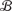was obtained by transforming the spike representation inside a sliding window of variable length: the length of the window depended on the category of the actual pattern. Then,was read out with time windows of size τ. That is the case, for example, when addressing the information conveyed by inter-spike intervals of length >38 ms using words of length τ = 14.8 ms (Reich et al., 2000) and by patterns of length >104, >10, and >56 ms, using time windows up to 64, 3.2, and 16 ms, respectively (Reinagel and Reid, 2000; Eyherabide et al., 2008; Gaudry and Reinagel, 2008). Unlike the first approach, in this case the data processing inequality does not apply, sinceτ is not a function of Rτ. Therefore, I(S; Bτ) can be larger or smaller than I(S; Rτ). However, when τ → ∞,τ = Bτ, so asymptotically, both approaches coincide.

### 4.4 The Role of Synergy and Redundancy in the Search for Relevant Response Aspects

One of the main goals of the analysis of the neural code is to identify the response aspects that are relevant to information transmission. In this context, two important questions arise: how relevant the chosen aspects are, and how autonomously they stand. Their relevance to information transmission is assessed with information-theoretical measures, as exemplified here with the category and time information (see Section 4.2). Their autonomy refers to whether each aspect transmits information by itself or not, and whether the transmitted information is shared by other aspects or not. The degree of autonomy is assessed by quantifying the synergy/redundancy term (ΔSR) between the different aspects.

The concept of synergy/redundancy entails the comparison between the effect of the whole and the sum of the individual effects of the constituent parts. The concept requires the constituent parts to be univocally determined by the whole, as well as the whole to be completely determined given its constituent parts. In other words, the whole and the constituent parts must be related through a bijective function. In neuroscience, the synergy/redundancy between groups of neurons has been addressed by comparing the information carried by the group of neurons (the whole) and the sum of the information of each and every neuron from the group (the constituent parts) (Brenner et al., 2000; Schneidman et al., 2003). As a result, ΔSR can be interpreted as a trade-off between synergy and redundancy (Schneidman et al., 2003).

Intuitively, the presence of synergy (ΔSR > 0) between two aspects indicates that, for many responses, the aspects must be read out simultaneously in order to obtain information about the stimulus. For some specific responses, however, one of the aspects may be enough to identify the stimulus. But on average, aspects cooperate. On the other hand, the presence of redundancy (ΔSR < 0) indicates that, for many responses, the information conveyed by both aspects overlaps. Therefore, some of the information that can be extracted from one aspect taken alone can also be extracted from the other aspect taken alone. There might still be a few individual responses for which it is necessary to read both aspects simultaneously to obtain information about the stimulus. But on average, messages tend to be replicated in the different aspects.

In the absence of synergy/redundancy (ΔSR = 0), the aspects might or might not be independent and conditionally independent given the stimulus (Nirenberg and Latham, 2003; Schneidman et al., 2003). If they are, then both aspects are fully autonomous. However, if they are not, then synergy and redundancy coexist. Some responses might require the simultaneous read out of both aspects. However, for other responses, at least one of the individual aspects might be enough to obtain information about the stimulus. In this case, by considering both aspects separately, one cannot recover the entire encoded information.

### 4.5 Applications

The main ideas in this paper can also be extended to encompass any neuron response aspects, different from pattern timing and pattern category. In particular, they allow us to analyze the information conveyed by different types of patterns and the synergy/redundancy between them, extending the formalism derived in Eyherabide et al. (2008). In addition, aspects may also be defined in continuous time since (Mackay and McCulloc, 1952). Even more, any neural population response can be represented as a single sequence of colored spikes, each color indicating the neuron that fired the spike (Brown et al., 2004). Therefore, single neuron codes and population codes can be analyzed under the same formalism.

For example, during the last decades, many studies have focused on assessing whether different neurons transmit information about different stimulus aspects (Gawne et al., 1996; Denning and Reinagel, 2005; Eyherabide et al., 2008). To that aim, different neurons (and different neural response aspects) have been interpreted as information channels (Dan et al., 1998; Montemurro et al., 2008; Krieghoff et al., 2009), often addressing whether they constitute independent channels of information (see Section 3.7). However, these studies have focused on whether the two aspects (or neurons) are independent and conditionally independent given the stimulus (Gawne and Richmond, 1993; Schneidman et al., 2003). Indeed, in this case, the response aspects constitute independent channels of information. However, these conditions do not identify which stimulus aspects are encoded by different neurons, nor they guarantee that they are independent.

To gain insight on the relation between stimulus and response aspects, we determine whether the neuron constitutes a canonical feature extractor and/or a canonical feature interpreter. For independent channels of information, the response aspects are canonical feature extractors, canonical feature interpreters, and also independent and conditionally independent given the stimulus. However, none of these conditions can be derived from the other. In effect, a canonical feature extractor or a canonical feature interpreter may or may not exhibit synergy or redundancy between the time and category information (see Section 3.7 and Appendix H). Moreover, even if T and C are independent and conditionally independent given the stimulus, T (C) may still convey information about SC (ST) once the information about ST (SC) has been read out. Formally, each of the equalities defining a canonical feature extractor or a canonical feature interpreter constitutes a relation between one aspect of the stimulus and one aspect of the response. Such relations cannot be derived from the independence or conditional independence between two aspects of the response. For the same reason, the what and the when are not guaranteed to be independent aspects.

Finally, the analysis performed in this work relies on the mutual information between the stimulus and different aspects of neural response, and thus it is related to both the encoding operation and the decoding operation (Shannon, 1948; Brown et al., 2004; Nelken and Chechik, 2007). Indeed, the mutual information is symmetric by definition (Cover and Thomas, 1991). Therefore, one can interpret the information between the stimulus and a particular aspect of the neural response from both points of view, characterizing both how the stimulus is encoded into a specific response aspect (Reich et al., 2000; Reinagel and Reid, 2000; Nelken et al., 2005; Eyherabide et al., 2008; Gollisch and Meister, 2008) and what can be inferred about the stimulus from a decoder that only decodes that specific aspect. To this aim, an explicit representation of each response aspect is needed, for example, as defined in Section 2.1. Such representations are not always available, as for example, in studies assessing the role of correlations (see Section 4.2).

## Conflict of Interest Statement

The authors declare that the research was conducted in the absence of any commercial or financial relationships that could be construed as a potential conflict of interest.

## Acknowledgments

This work was supported by the Alexander von Humboldt Foundation, the Consejo de Investigaciones Científicas y Técnicas, the Agencia de Promoción Científica y Tecnológica of Argentina, and Comisión Nacional de Energía Atómica.

## References

Abeles, M., and Gat, I. (2001). Detecting precise firing sequences in experimental data. J. Neurosci. Methods 107, 141–154.

Alitto, H. J., Weyand, T. H., and Usrey, W. M. (2005). Distinct properties of stimulus-evoked bursts in the lateral geniculate nucleus. J. Neurosci. 25, 514–523.

Apostol, T. M. (1990). Modular Functions and Dirichlet Series in Number Theory, 2nd Edn. New York: Springer-Verlag.

Benda, J., Longtin, A., and Maler, L. (2005). Spike-frequency adaptation separates transient communication signals from background oscillations. J. Neurosci. 25, 2312–2321.

Borst, A., and Theunissen, F. E. (1999). Information theory and neural coding. Nat. Neurosci. 2, 947–957.

Brenner, N., Strong, S. P., Korbele, R., Bialek, W., and de Ruyter van Steveninck, R. R. (2000). Synergy in a neural code. Neural. Comput. 12, 1531–1552.

Brown, E. N., Kass, R. E., and Mitra, P. P. (2004). Multiple neural spike train data analysis: state-of-the-art and future challenges. Nat. Neurosci. 7, 456–461.

Chase, S. M., and Young, E. D. (2007). First-spike latency information in single neurons increases when referenced to population onset. Proc. Natl. Acad. Sci. U.S.A. 104, 5175–5180.

Cover, T. M., and Thomas, J. A. (1991). Elements of Information Theory. New York: Wiley.

Dan, Y., Alonso, J. M., Usrey, W. M., and Reid, R. C. (1998). Coding of visual information by precisely correlated spikes in the lateral geniculate nucleus. Nat. Neurosci. 1, 501–507.

Dayan, P., and Abbott, L. F. (2001). Theoretical Neuroscience: Computational and Mathematical Modeling of Neural Systems. Cambridge, MA: MIT Press.

DeBusk, B. C., DeBruyn, E. J., Snider, R. K., Kabara, J. F., and Bonds, A. B. (1997). Stimulus-dependent modulation of spike burst length in cat striate cortical cells. J. Neurophysiol. 78, 199–213.

Denning, K. S., and Reinagel, P. (2005). Visual control of bursts priming in the anesthetized lateral geniculate nucleus. J. Neurosci. 25, 3531–3538.

Eyherabide, H. G., Rokem, A., Herz, A. V. M., and Samengo, I. (2008). Burst firing is a neural code in an insect auditory system. Front. Comput. Neurosci. 2:3. doi: 10.3389/neuro.10.003.2008.

Eyherabide, H. G., Rokem, A., Herz, A. V. M., and Samengo, I. (2009). Bursts generate a non-reducible spike pattern code. Front. Neurosci. 3:1. doi: 10.3389/neuro.01.002.2009.

Eyherabide, H. G., and Samengo, I. (2010). The information transmitted by spike patterns in single neurons. J. Physiol. (Paris) 104, 147–155.

Fellous, J. M., Tiesinga, P. H. E., Thomas, P. J., and Sejnowski, T. J. (2004). Discovering spike patterns in neuronal responses. J. Neurosci. 24, 2989–3001.

Foffani, G., Morales, M. L., and Aguilar, J. (2009). Spike timing, spike count, and temporal information for the discrimination of tactile stimuli in the rat ventrobasal complex. J. Neurosci. 29, 5964–5973.

Furukawa, S., and Middlebrooks, J. C. (2002). Cortical representation of auditory space: information-bearing features of spike patterns. J. Neurophysiol. 87, 1749–1762.

Gaudry, K. S., and Reinagel, P. (2008). Information measure for analyzing specific spiking patterns and applications to lgn bursts. Netw. Comput. Neural Syst. 19, 69–94.

CrossRef Full Text

Gawne, T. J., Kjaer, T. W., and Richmond, B. J. (1996). Latency: Another potential code for feature binding in striate cortex. J. Neurophysiol. 76, 1356–1360.

Gawne, T. J., and Richmond, B. J. (1993). How independent are the messages carried by adjacent inferior temporal cortical neurons? J. Neurosci. 73, 2758–2771.

Gollisch, T., and Meister, M. (2008). Rapid neural coding in the retina with relative spike latencies. Science 319, 1108–1111.

Gütig, R., and Sompolinsky, H. (2006). The tempotron: a neuron that learns spike timing-based decisions. Nat. Neurosci. 9, 420–428.

Hopfield, J. J. (1995). Pattern recognition computation using action potential timing for stimulus representation. Nature 376, 33–36.

Krahe, R., and Gabbiani, F. (2004). Burst firing in sensory systems. Nat. Rev. Neurosci. 5, 13–23.

Krieghoff, V., Brass, M., Prinz, W., and Waszak, F. (2009). Dissociating what and when of intentional actions. Front. Hum. Neurosci. 3.

Lisman, J. E. (1997). Bursts as a unit of neural information: making unreliable synapses reliable. Trends Neurosci. 20, 38–43.

Luna, R., Hernández, A., Brody, C. D., and Romo, R. (2005). Neural codes for perceptual discrimination in primary somatosensory cortex. Nat. Neurosci. 8, 1210–1219.

Mackay, D. M., and McCulloc, W. S. (1952). The limiting information capacity of a neuronal link. Bull. Math. Biophys. 14, 127–135.

CrossRef Full Text

Mancuso, K., Hauswirth, W. W., Li, Q., Connor, T. B., Kuchenbecker, J. A., Mauck, M. C., Neitz, J., and Neitz, M. (2009). Gene therapy for red-green colour blindness in adult primates. Nature 461, 784–788.

Marsat, G., Proville, R. D., and Maler, L. (2009). Transient signals trigger synchronous bursts in an identified population of neurons. J. Neurophysiol. 102, 714–723.

Martinez-Conde, S., Macknik, S. L., and Hubel, D. H. (2000). Microsaccadic eye movements and firing of single cells in the striate cortex of macaque monkeys. Nat. Neurosci. 3, 251–258.

Martinez-Conde, S., Macknik, S. L., and Hubel, D. H. (2002). The function of bursts of spikes during visual fixation in the awake primate lateral geniculate nucleus and primary visual cortex. Proc. Natl. Acad. Sci. U.S.A. 99, 13920–13925.

Montemurro, M. A., Panzeri, S., Maravall, M., Alenda, A., Bale, M. R., Brambilla, M., and Petersen, R. S. (2007). Role of precise spike timing in coding of dynamic vibrissa stimuli. J. Neurophysiol. 98, 1871–1882.

Montemurro, M. A., Rasch, M. J., Murayama, Y., Logothetis, N. K., and Panzeri, S. (2008). Phase-of-firing coding of natural visual stimuli in primary visual cortex. Curr. Biol. 18, 375–380.

Nádasdy, Z. (2000). Spike sequences and their consequences. J. Physiol. (Paris) 94, 505–524.

CrossRef Full Text

Nelken, I. (2008). Processing of complex sounds in the auditory system. Curr. Opin. Neurobiol. 18, 413–417.

Nelken, I., and Chechik, G. (2007). Information theory in auditory research. Hear. Res. 229, 94–105.

Nelken, I., Chechik, G., Mrsic-Flogel, T. D., King, A. J., and Schnupp, J. W. H. (2005). Encoding stimulus information by spike numbers and mean response time in primary auditory cortex. J. Comput. Neurosci. 19, 199–221.

Nemenman, I., Bialek, W., and de Ruyter van Steveninck, R. R. (2004). Entropy and information in neural spike trains: Progress on the sampling problem. Phys. Rev. E 69, 056111.

CrossRef Full Text

Nirenberg, S., Carcieri, S. M., Jacobs, A. L., and Latham, P. E. (2001). Retinal ganglion cells act largely as independent decoders. Nature 411, 698–701.

Nirenberg, S., and Latham, P. E. (2003). Decoding neuronal spike trains: How important are correlations. Proc. Natl. Acad. Sci. U.S.A. 100, 7348–7353.

Oswald, A. M. M., Doiron, B., and Maler, L. (2007). Interval coding. I. burst interspike intervals as indicators of stimulus intensity. J. Neurophysiol. 97, 2731–2743.

Panzeri, S., Senatore, R., Montemurro, M. A., and Petersen, R. S. (2007). Correcting for the sampling bias problem in spike train information measures. J. Neurophysiol. 98, 1064–1072.

Petersen, R. S., Panzeri, S., and Diamond, M. E. (2002). The role of individual spikes and spike patterns in population coding of stimulus location in rat somatosensory cortex. BioSystems 67, 187–193.

Poulos, D. A., Mei, J., Horch, K. W., Tuckett, R. P., Wei, J. Y., Cornwall, M. C., and Burgess, P. R. (1984). The neural signal for the intensity of a tactile stimulus. J. Neurosci. 4, 2016–2024.

Reich, D. S., Mechler, F., Purpura, K. P., and Victor, J. D. (2000). Interspike intervals, receptive fields, and information encoding in primary visual cortex. J. Neurosci. 20, 1964–1974.

Reinagel, P., Godwin, D., Sherman, S. M., and Koch, C. (1999). Encoding of visual information by lgn bursts. J. Neurophysiol. 81, 2558–2569.

Reinagel, P., and Reid, R. C. (2000). Temporal coding of visual information in the thalamus. J. Neurosci. 20, 5392–5400.

Reinagel, P., and Reid, R. C. (2002). Precise firing events are conserved across neurons. J. Neurosci. 22, 6837–6841.

Rice, J. A. (1995). Mathematical Statistics and Data Analysis, 2nd Edn. Belmont, CA: Duxbury Press, Wadsworth Publishing Company.

Rokem, A., Watzl, S., Gollisch, T., Stemmler, M., Herz, A. V. M., and Samengo, I. (2006). Spike-timing precision underlies the coding efficiency of auditory receptor neurons. J. Neurophysiol. 95, 2541–2552.

Rolen, S. H., and Caprio, J. (2007). Processing of bile salt odor information by single olfactory bulb neurons in the channel catfish. J. Neurophysiol. 97, 4058–4068.

Sabourin, P., and Pollack, G. S. (2009). Behaviorally relevant burst coding in primary sensory neurons. J. Neurophysiol. 102, 1086–1091.

Schneidman, E., Bialek, W., and Berry, M. J. (2003). Synergy, redundancy, and independence in population codes. J. Neurosci. 23, 11539–11553.

Shannon, C. E. (1948). A mathematical theory of communication. Bell Syst. Tech. J. 27, 379–423.

Strong, S. P., Koberle, R., de Ruyter van Steveninck, R. R., and Bialek, W. (1998). Entropy and information in neural spike trains. Phys. Rev. Lett. 80, 197–200.

CrossRef Full Text

Theunissen, F., and Miller, J. P. (1995). Temporal encoding in nervous systems: a rigorous definition. J. Comput. Neurosci. 2, 149–162.

Tsujishita, T. (1995). On triple mutual information. Adv. Appl. Math. 16, 269–274.

CrossRef Full Text

Victor, J. D. (2002). Binless strategies for estimation of information in neural data. Phys. Rev. E 051903.

CrossRef Full Text

Keywords: patterns, neural code, sensory encoding, information theory, redundancy, synergy, stimulus features, feature extractor

Citation: Eyherabide HG and Samengo I (2010) Time and category information in pattern-based codes. Front. Comput. Neurosci. 4:145. doi: 10.3389/fncom.2010.00145

Received: 29 May 2010; Accepted: 22 October 2010;
Published online: 23 November 2010.

Edited by:

Israel Nelken, Hebrew University, Israel

Reviewed by:

Stefano Panzeri, Italian Institute of Technology, Italy
Jakob H. Macke, University College London, UK

Copyright: © 2010 Eyherabide and Samengo. This is an open-access article subject to an exclusive license agreement between the authors and the Frontiers Research Foundation, which permits unrestricted use, distribution, and reproduction in any medium, provided the original authors and source are credited.

*Correspondence: Hugo Gabriel Eyherabide, Centro Atómico Bariloche, San Carlos de Bariloche, 8400 Rìo Negro, Argentina. e-mail: eyherabh@ib.cnea.gov.ar